# Catch up on the latest AI articles# An Offline Meta-RL Framework That Allows Robots To Quickly Adapt To Unknown Complex Insertion Tasks Using Data Collected In The Past!

3 main points
✔️ We propose an offline meta-RL framework that can solve complex insertion tasks.
✔️ By using demonstration and offline data, we can quickly adapt to unknown tasks.
✔️ Achieve 100% success rate in 30 minutes or less for all 12 tasks by finetuning even when the tasks at training and testing are very different

(Submitted on 21 Mar 2021 (v1), last revised 31 Jul 2021 (this version, v4))

Subjects: Artificial Intelligence (cs.AI); Robotics (cs.RO)code：The images used in this article are from the paper, the introductory slides, or were created based on them.

## first of all

In recent years, we have shown in various studies that reinforcement learning can achieve a high success rate in a part insertion task using an actual robot by learning the policy. In particular, we have shown that the method introduced in this article can solve a very complex insertion task with a high success rate by using a small amount of demonstration, reinforcement learning, and some innovations. However, if we want to solve an insertion task with different components, we have to relearn the method from scratch. In this article, we introduce a paper called Offline Meta-Reinforcement Learning for Industrial Insertion, which solves such a problem.

Recently, meta-RL has been attracting attention as a reinforcement learning method that can instantly adapt to unknown data. However, meta-RL requires very time-consuming online meta-RL training, which is difficult to perform in the real world. Therefore, in this paper, we proposed a method that can adapt to unknown parts instantly by performing online meta-RL using various offline datasets collected beforehand, as shown in the figure below, and then performing online finetuning. Now, I will explain the details of this paper.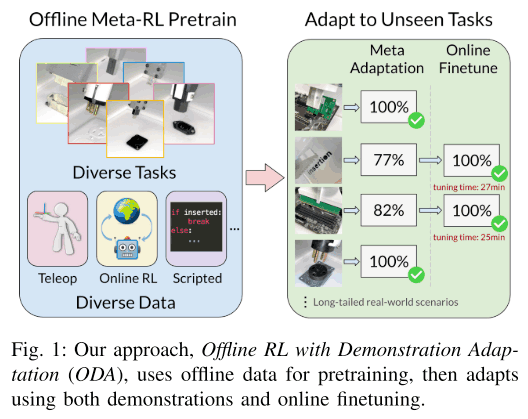## technique

In this chapter, we explain the proposed method in detail. First, we train an adaptive policy by meta-training with offline RL. The data set consists of demonstrations of tasks using various components collected in advance and offline data (e.g., data stored in the replay buffer from previous training). The adaptive policy is then used for the unknown task, where a small number of demonstrations and online RL finetuning are used to obtain the final policy. In this way, it is safer and easier to adapt a policy to an unknown task than to adapt a policy learned in the past to an unknown task by online RL.

The policy used in this method consists of a policy network $\pi(a|s, z)$ and an encoder $q_{\phi}(z|c)$, where $s$ represents the current state and $z$ represents the latent code representing the task. Thus, the encoder extracts the important information necessary for how to execute the task from the task demonstration and puts it into the latent code. This method is similar to the PEARL and MELD methods, but in the part where the latent code is obtained, the latent code is obtained from the demonstration, while those methods use the online experience.

Then, when adapting to an unknown task, a small amount of demonstration is given as the context of the task, and the latent code $z\sim q(z|c)$ can be inferred based on it, and policy can be used for the unknown task. However, if the unknown task is very different from the task used in meta-training, it is difficult to estimate $z$ such that policy can solve the unknown task. In such cases, we use online RL to finetuning the policy. The experiments in this paper show that this finetuning takes about 5~10 minutes.

Now let's discuss each of these steps in more detail.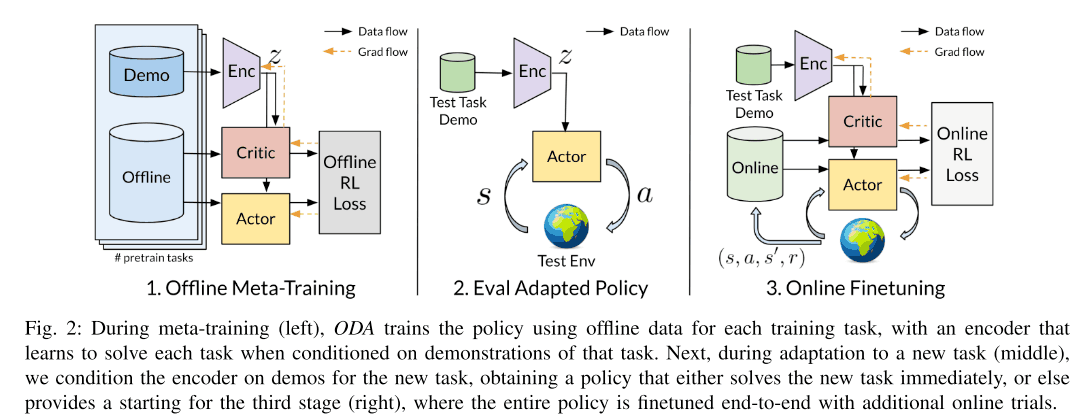### Contextual Meta-Learning and Demonstrations

As explained above, unlike previous methods such as PEARL and MELD, the proposed method in this paper learns encoder $q_{\phi}(z|c)$ using demonstration as the task context. The output latent code $z$ is then passed to policy $\pi_{\theta}(a|s, z)$. The advantage of accepting this demonstration is that we can suppress the train-test distribution shift, which is performance degradation due to the difference in distribution between training and testing. This is because the data collected for the unknown task at test time and the offline data used at training time are collected from different policies, which can easily cause distribution shifts. This problem is solved by using demonstration.

During training, encoder $q_{\phi}(z|c)$ and policy $\pi_{\theta}(a|s, z)$ are trained to maximize the performance of the meta-training task simultaneously. In addition, two types of data are used during training: one is the demonstration, and the other consists of offline data used to update the actor-critic. This offline data corresponds to the data stored in the replay buffer of the previously trained task. The encoder also adds KL divergence loss to the latent code $z$ so that it contains as little unnecessary information as possible. These detailed algorithms are illustrated by the algorithm diagram below.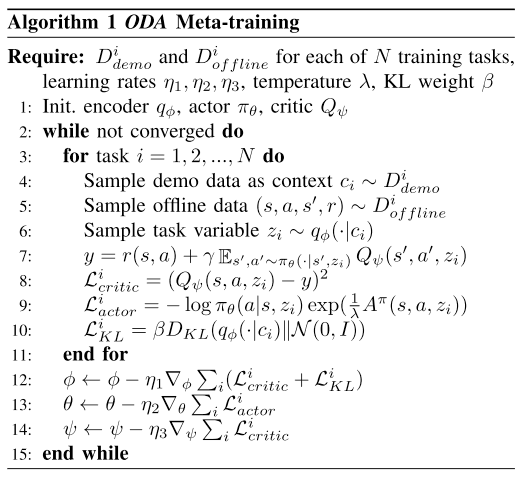### Offline & Online Reinforcement Learning

Since this method requires meta-training with offline data, a suitable algorithm for offline RL is required, and fine-tuning is also required. Since fine-tuning is also required afterward, we use a method called Advantage-Weighted Actor-Critic (AWAC), which maximizes the reward and learns a policy to keep the data distribution $\pi_{\beta}$ as shown in the following equation. AWAC learns the policy to maximize the reward and to stay close to the distribution of data $\pi_{\beta}$, as in the following equation.

$$\theta^{\star}=\arg \max \mathbb{E}_{\mathbf{s} \sim \mathcal{D}}\mathbb{E}_{\pi_{\theta}(\mathbf{a} \mid \mathbf{s})}\left[Q_{\phi}(\mathbf{s}, \mathbf{a})}right] \text { s.t.} D_{K L}\left(\pi_{\theta} \| \pi_{\beta}\right) \leq \epsilon$$

This is approximated using the Lagrangian by a weighted maxlmum likelihood as follows

$$\theta^{\star}=\underset{\theta}{\arg \max } \mathbb{E}_{\mathbf{s}, \mathbf{a} \sim \beta}\left[\log \pi_{\theta}(\mathbf{a} \mid \mathbf{s}) \exp \left(A^{\pi}(\mathbf{s}, \mathbf{a})\right)\right]$$

where $A^{\pi}(\mathbf{s}, \mathbf{a})=Q_{\phi}(\mathbf{s}, \mathbf{a})-E_{\mathbf{a} \sim \pi_{\theta}(\mathbf{a} \mid \mathbf{s})}\left[Q_{\phi}(\mathbf{s}, \mathbf{a})\right]$.

Using this technique, after meta-training, fine-tuning is performed when necessary to maximize the performance of the unknown task. The specific algorithm is as follows.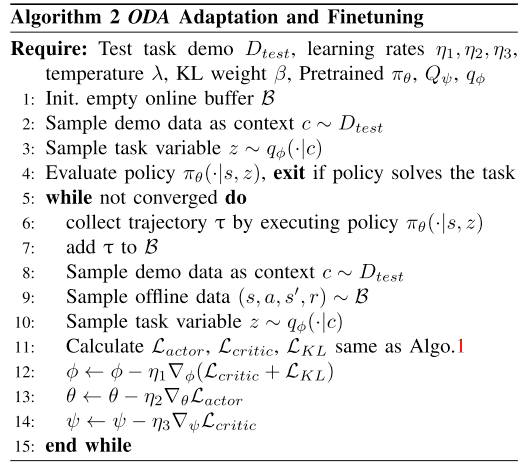## experiment

In this paper, we evaluated the proposed method on 12 unknown tasks and answered the following four questions.

1. whether it is good compared to normal offline RL
2. whether finetuning can be used to quickly adapt to unknown tasks that are very different from the task used for training
3. whether it can adapt to more difficult tasks such as the one shown in the figure below
4. whether meta-adaptation can be improved by using more data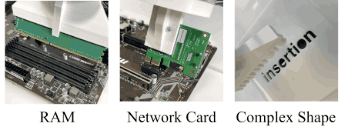The KUKA iiwa7 robot is used in the experiments, and the TCP pose, velocity, and force-torque (wrench) acting on the tooltip are given as observations. At the beginning of each episode, the robot is given a noise sampled from a uniform distribution $U$[-1mm, 1mm].

As an offline dataset, we collected data from 11 different plug and socket pairs as shown in the figure below. We used the data stored in the replay buffer of a previous paper using DDPGfD as offline data. We also used the demonstrations that were used for training in the same way for training this method.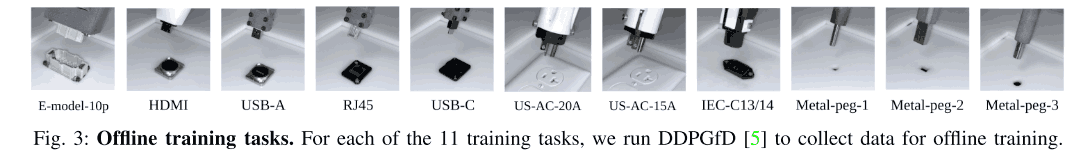In this experiment, we evaluated how well the trained encoder could adapt to an unknown task using demonstrations. In this experiment, we evaluated the encoder on the nine test tasks shown in the figure below and collected 20 demonstrations for each task. In this experiment, we compared the performance of the proposed method with that of a regular offline RL (AWAC) training method, and the results are shown in the table below. From the figure below, we can see that overall the proposed method can achieve a higher success rate.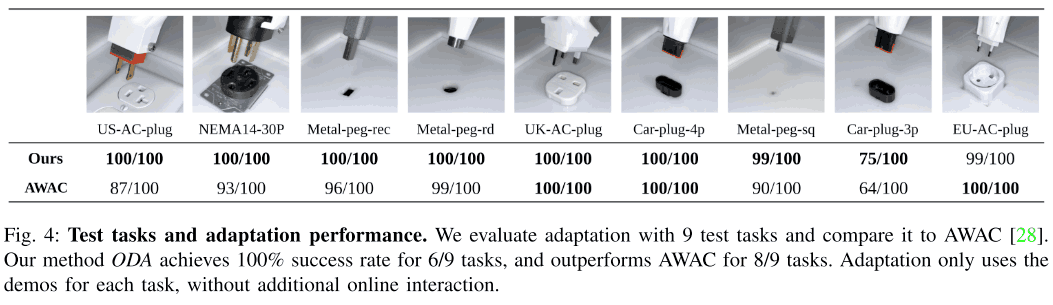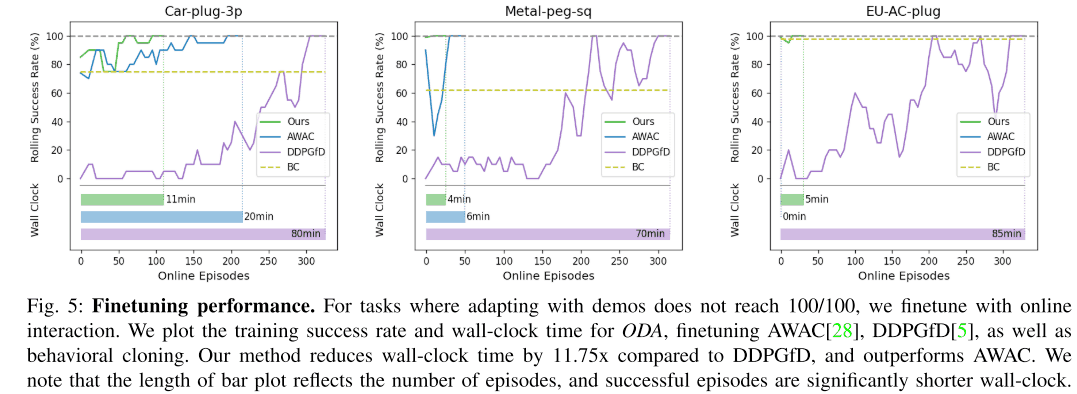### For the more difficult Out-of-Distribution tasks

But what about more complex tasks? In this paper, we conducted experiments on three tasks as shown below. As shown in the figure below, we achieved a 100% success rate for Network Card without fine-tuning, and a 70-80% success rate for the other tasks. What is especially important is that there is no damage to the motherboard during this fine-tuning.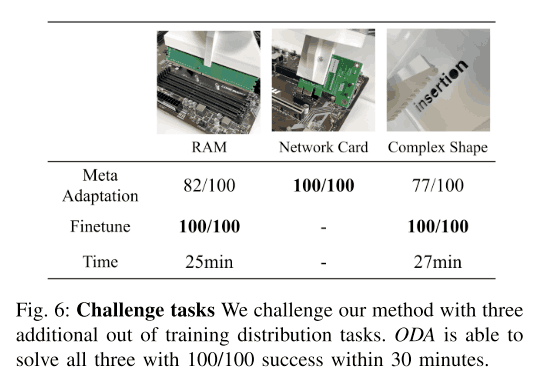### Relationship between the number of tasks and the success rate after adaptation

Finally, we examined the relationship between the task and the success rate of the unknown task after adaptation. In this experiment, we did not use fine-tuning, but an only demonstration. The graph below shows the results. As shown in the figure below, the success rate is close to the maximum when the number of tasks is six, and the success rate decreases significantly when the number of tasks is less than six. Thus, it is clear that collecting training data for a large number of tasks has a significant impact on performance.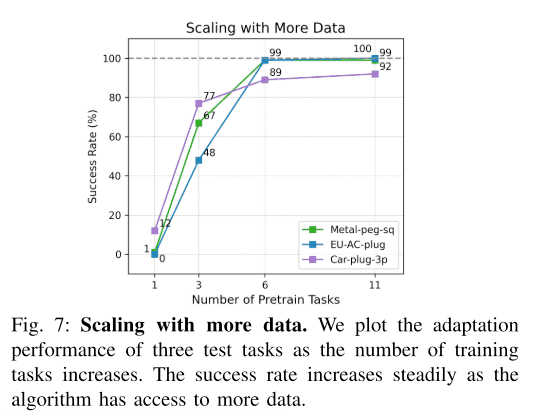## summary

In the paper described in this article, we concentrate on solving only one task and have to relearn from scratch when solving other insertion tasks. In this paper, we propose a method that solves this problem. In this method, a low-dimensional state is used as the observation of the policy, but it may be a key to solving the problem with images as input.

If you have any suggestions for improvement of the content of the article,